# 信号平滑处理

### 目的

```load bostemp days = (1:31*24)/24; plot(days, tempC) axis tight ylabel('Temp (\circC)') xlabel('Time elapsed from Jan 1, 2011 (days)') title('Logan Airport Dry Bulb Temperature (source: NOAA)')```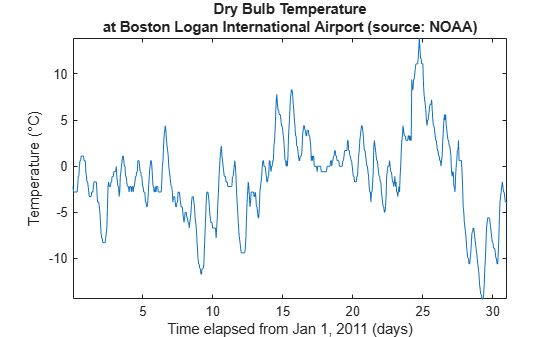### 一种移动平均滤波器

```hoursPerDay = 24; coeff24hMA = ones(1, hoursPerDay)/hoursPerDay; avg24hTempC = filter(coeff24hMA, 1, tempC); plot(days,[tempC avg24hTempC]) legend('Hourly Temp','24 Hour Average (delayed)','location','best') ylabel('Temp (\circC)') xlabel('Time elapsed from Jan 1, 2011 (days)') title('Logan Airport Dry Bulb Temperature (source: NOAA)')```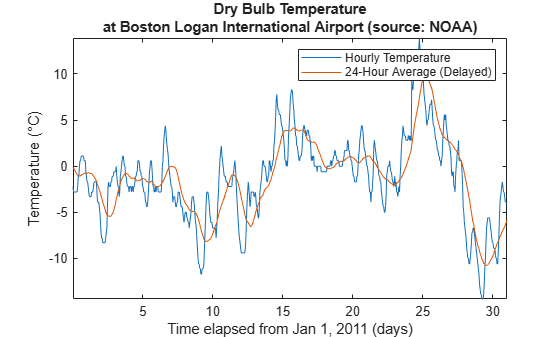### 滤波器延迟

```fDelay = (length(coeff24hMA)-1)/2; plot(days,tempC, ... days-fDelay/24,avg24hTempC) axis tight legend('Hourly Temp','24 Hour Average','location','best') ylabel('Temp (\circC)') xlabel('Time elapsed from Jan 1, 2011 (days)') title('Logan Airport Dry Bulb Temperature (source: NOAA)')```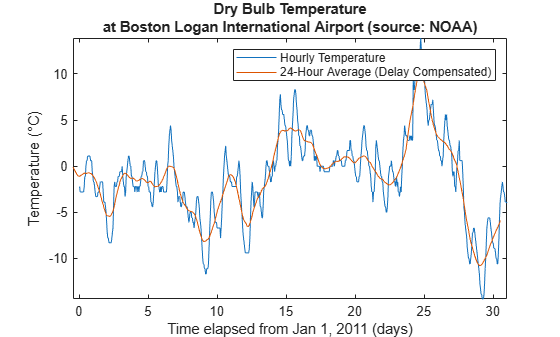### 提取平均差异

```figure deltaTempC = tempC - avg24hTempC; deltaTempC = reshape(deltaTempC, 24, 31).'; plot(1:24, mean(deltaTempC)) axis tight title('Mean temperature differential from 24 hour average') xlabel('Hour of day (since midnight)') ylabel('Temperature difference (\circC)')```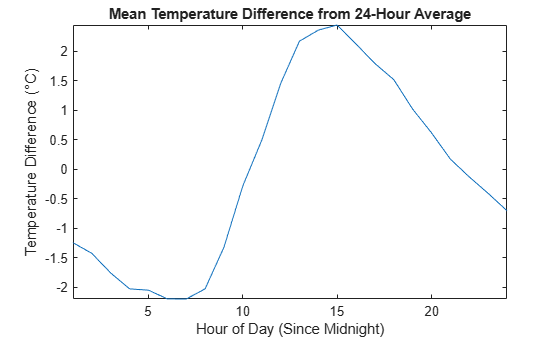### 提取峰值包络

```[envHigh, envLow] = envelope(tempC,16,'peak'); envMean = (envHigh+envLow)/2; plot(days,tempC, ... days,envHigh, ... days,envMean, ... days,envLow) axis tight legend('Hourly Temp','High','Mean','Low','location','best') ylabel('Temp (\circC)') xlabel('Time elapsed from Jan 1, 2011 (days)') title('Logan Airport Dry Bulb Temperature (source: NOAA)')```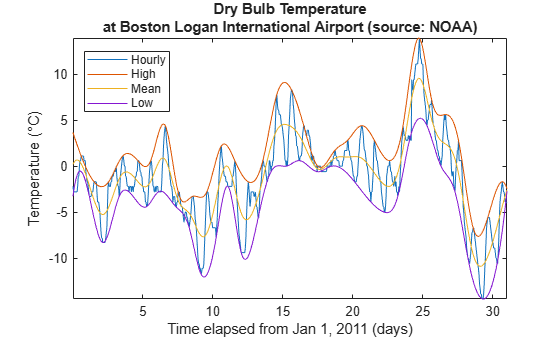### 加权移动平均滤波器

```h = [1/2 1/2]; binomialCoeff = conv(h,h); for n = 1:4 binomialCoeff = conv(binomialCoeff,h); end figure fDelay = (length(binomialCoeff)-1)/2; binomialMA = filter(binomialCoeff, 1, tempC); plot(days,tempC, ... days-fDelay/24,binomialMA) axis tight legend('Hourly Temp','Binomial Weighted Average','location','best') ylabel('Temp (\circC)') xlabel('Time elapsed from Jan 1, 2011 (days)') title('Logan Airport Dry Bulb Temperature (source: NOAA)')```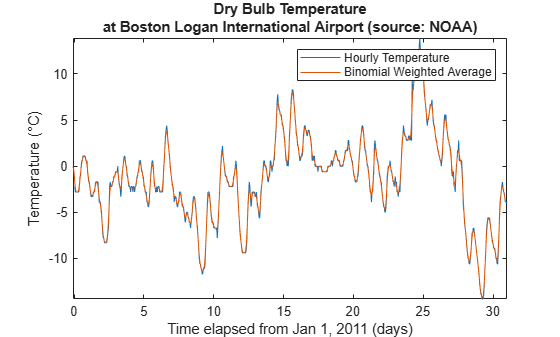```alpha = 0.45; exponentialMA = filter(alpha, [1 alpha-1], tempC); plot(days,tempC, ... days-fDelay/24,binomialMA, ... days-1/24,exponentialMA) axis tight legend('Hourly Temp', ... 'Binomial Weighted Average', ... 'Exponential Weighted Average','location','best') ylabel('Temp (\circC)') xlabel('Time elapsed from Jan 1, 2011 (days)') title('Logan Airport Dry Bulb Temperature (source: NOAA)')```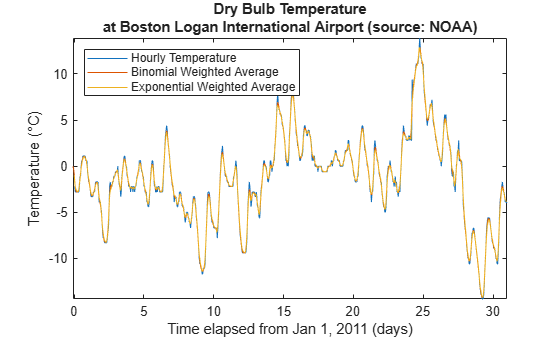`axis([3 4 -5 2])`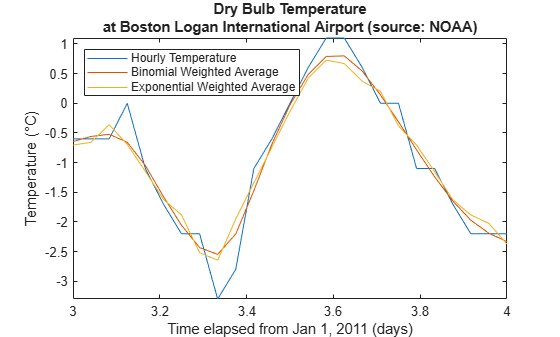### Savitzky-Golay 滤波器

```cubicMA = sgolayfilt(tempC, 3, 7); quarticMA = sgolayfilt(tempC, 4, 7); quinticMA = sgolayfilt(tempC, 5, 9); plot(days,[tempC cubicMA quarticMA quinticMA]) legend('Hourly Temp','Cubic-Weighted MA', 'Quartic-Weighted MA', ... 'Quintic-Weighted MA','location','southeast') ylabel('Temp (\circC)') xlabel('Time elapsed from Jan 1, 2011 (days)') title('Logan Airport Dry Bulb Temperature (source: NOAA)') axis([3 5 -5 2])```### 重采样

```load openloop60hertz fs = 1000; t = (0:numel(openLoopVoltage)-1) / fs; plot(t,openLoopVoltage) ylabel('Voltage (V)') xlabel('Time (s)') title('Open-loop Voltage Measurement')```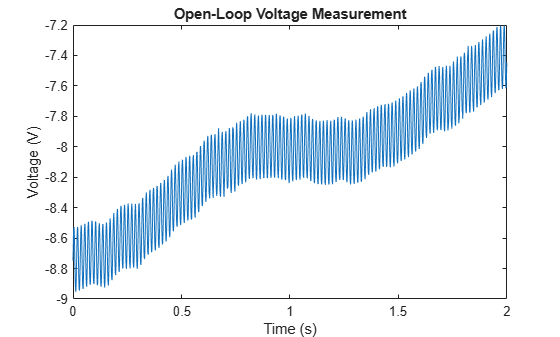```plot(t,sgolayfilt(openLoopVoltage,1,17)) ylabel('Voltage (V)') xlabel('Time (s)') title('Open-loop Voltage Measurement') legend('Moving average filter operating at 58.82 Hz', ... 'Location','southeast')```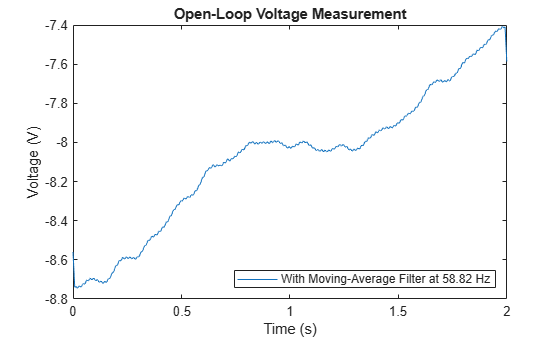```fsResamp = 1020; vResamp = resample(openLoopVoltage, fsResamp, fs); tResamp = (0:numel(vResamp)-1) / fsResamp; vAvgResamp = sgolayfilt(vResamp,1,17); plot(tResamp,vAvgResamp) ylabel('Voltage (V)') xlabel('Time (s)') title('Open-loop Voltage Measurement') legend('Moving average filter operating at 60 Hz', ... 'Location','southeast')```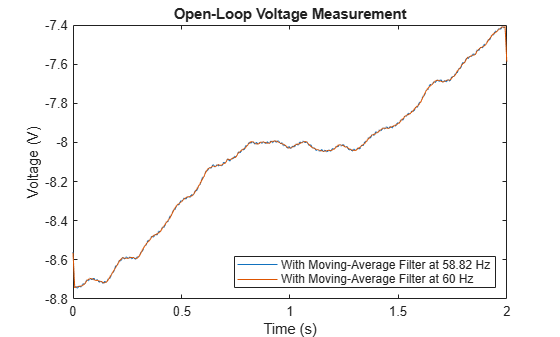### 中位数滤波器

```load clockex yMovingAverage = conv(x,ones(5,1)/5,'same'); ySavitzkyGolay = sgolayfilt(x,3,5); plot(t,x, ... t,yMovingAverage, ... t,ySavitzkyGolay) legend('original signal','moving average','Savitzky-Golay')```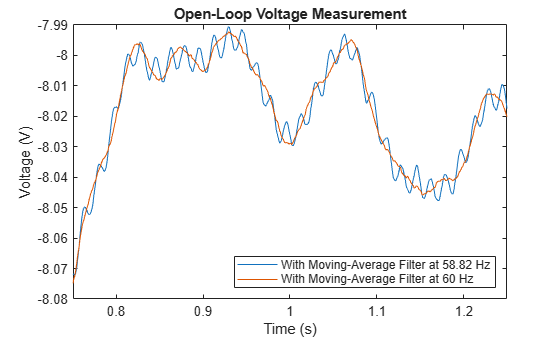```yMedFilt = medfilt1(x,5,'truncate'); plot(t,x, ... t,yMedFilt) legend('original signal','median filter')```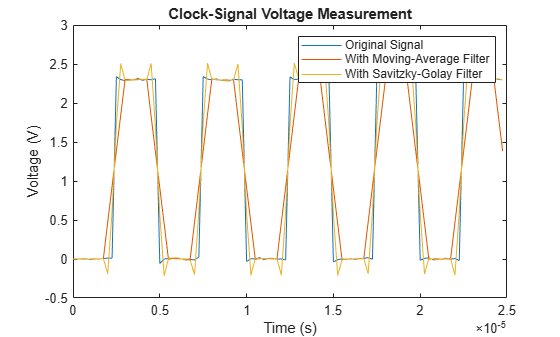### 通过 Hampel 滤波器去除离群值

```load train y(1:400:end) = 2.1; plot(y)```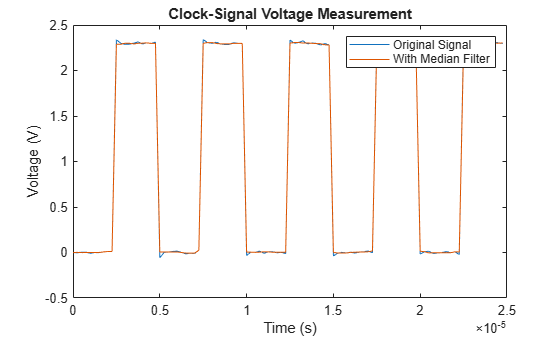```hold on plot(medfilt1(y,3)) hold off legend('original signal','filtered signal')```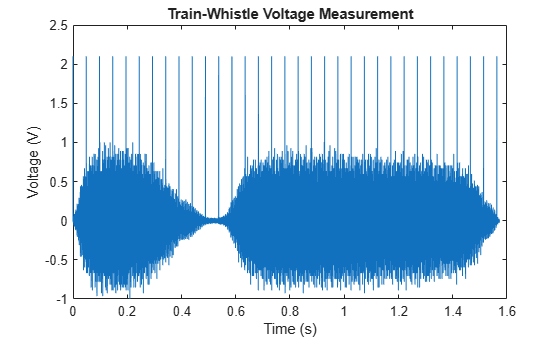```hampel(y,13) legend('location','best')```